# Texas Go Math Grade 1 Lesson 5.5 Answer Key Subtract All or Zero

Refer to our Texas Go Math Grade 1 Answer Key Pdf to score good marks in the exams. Test yourself by practicing the problems from Texas Go Math Grade 1 Lesson 5.5 Answer Key Subtract All or Zero.

## Texas Go Math Grade 1 Lesson 5.5 Answer Key Subtract All or Zero

Essential Question
What happens when you subtract 0 from a number?

Explore
Useto show the problem. Draw the. Write the numbers.

___ – ___ = ____

___ – ___ = ____
Explanation: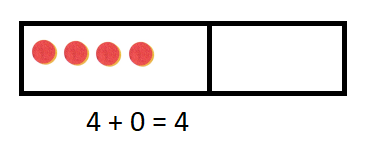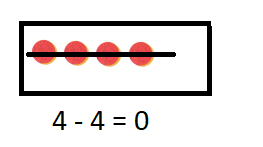4 + 0 = 4
4 – 4 = 0

For The Teacher

• Read the following problem. 4 toys are on the shelf. 0 toys are taken off. How many toys are on the shelf? Then read the following problem. 4 toys are on the shelf. 4 toys are taken off. How many toys are there now?Explanation:
4 + 0 = 4
4 – 4 = 0
There are 0 toys now.

Math Talk
Explanation:
In each case the answer is correct according to given question.
Yes the first answer also make sense

Model and Draw

When you subtract zero, how many are left?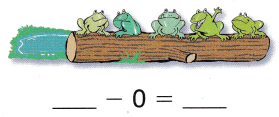When you subtract all, how many are left?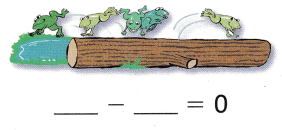If we subtract all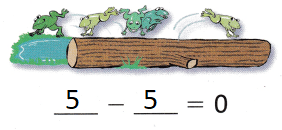Explanation:
4 – 0 = 4
4 – 4 = 0
The number subtracted with the 0 the answer remains itself
When a number subtracted by itself the answer is 0

Share and Show

Use the picture to complete the subtraction sentence.

Question 1.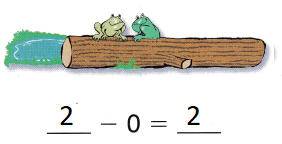Explanation:
2 – 0 = 2
The number subtracted with the 0 the answer remains itself
When a number subtracted by itself the answer is 0

Question 2.Explanation:
2 – 2 = 0
The number subtracted with the 0 the answer remains itself
When a number subtracted by itself the answer is 0

Question 3.Explanation:
6 – 6 = 0
The number subtracted with the 0 the answer remains itself
When a number subtracted by itself the answer is 0

Question 4.Explanation:
6 – 0 = 6
The number subtracted with the 0 the answer remains itself
When a number subtracted by itself the answer is 0

Question 5.Explanation:
4 – 0 = 4
The number subtracted with the 0 the answer remains itself
When a number subtracted by itself the answer is 0

Question 6.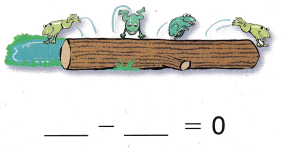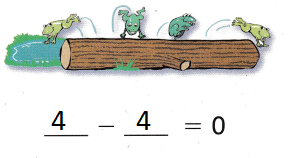Explanation:
4 – 4 = 0
The number subtracted with the 0 the answer remains itself
When a number subtracted by itself the answer is 0

Problem Solving

Complete the subtraction sentence.

Question 7.

3 – 0 = ___
Explanation:
3 – 0 = 3
The difference of  3 and 0 is 3

Question 8.

____ = 6 – 0
Explanation:
6 – 0 = 6
The difference of 6 and 0 is 6

Question 9.

7 – ___ = 7
7 – 0 = 7
Explanation:
The difference of  7 and 0 is 7

Question 10.

8 – 8 = ___
8 – 8 = 0
Explanation:
The difference of 8 and 8 is 0

Problem Solving

Write or draw to solve.

Question 11.
There are 6 cups on the table. 4 are blue and the rest are yellow. How many cups are yellow?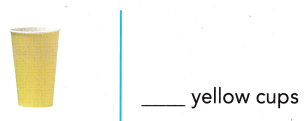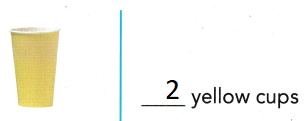Explanation:
4 + 2 = 6
6 – 2 = 4
The number subtracted with the 0 the answer remains itself
When a number subtracted by itself the answer is 0

Question 12.
H.O.T. Kevin has 3 fewer leaves than Sandy. Sandy has 3 leaves. How many leaves does Kevin have?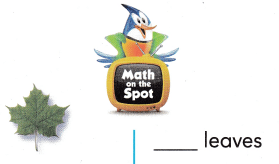Explanation:
3 – 3 = 0
0 leaves that Kevin have
The number subtracted with the 0 the answer remains itself
When a number subtracted by itself the answer is 0

Question 13.
Multi-Step Jared has 8 pictures. He gives some to Wendy. He gives 2 to Josh. Now Jared has 0 pictures. How many did he give to Wendy?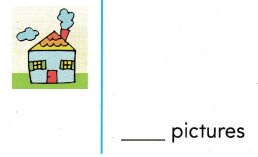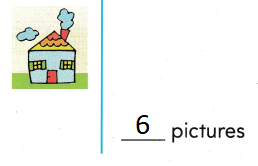Explanation:
2 + 6 = 8
8 – 8 = 0
6 he give to Wendy
The number subtracted with the 0 the answer remains itself
When a number subtracted by itself the answer is 0

Question 14.
Robbie moves 4 shells around. He takes 0 shells. What number sentence shows how many shells are left?
(A) 4 – 4 = 0
(B) 5 – 4 = 1
(C) 4 – 0 = 4
4 – 0 = 4
Explanation:
4 shells are left
The number subtracted with the 0 the answer remains itself
When a number subtracted by itself the answer is 0

Question 15.
There are 6 puppies at the park. 6 puppies go home. Which number sentence shows how many puppies are left?
(A) 6 – 6 = 0
(B) 6 – 1 = 5
(C) 6 – 0 = 6Explanation:
6 – 6 = 0
0 puppies are left
The number subtracted with the 0 the answer remains itself
When a number subtracted by itself the answer is 0

Question 16.
Analyze Jodie has 7 fewer fish than Marty. Marty has 7 fish. How many fish does Jodie have?
(A) 4
(B) 0
(C) 3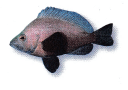Explanation:
7 – 7 = 0
Jodie have 0 fishes
The number subtracted with the 0 the answer remains itself
When a number subtracted by itself the answer is 0

Question 17.
Texas Test Prep What is the difference for 5 – 0?
(A) 5
(B) 0
(C) 1
Explanation:
5 – 0 = 5
The number subtracted with the 0 the answer remains itself
When a number subtracted by itself the answer is 0

Take Home Activity

• Have your child explain how 4 – 4 and 4 – 0 are different.
Explanation:
4 – 4 = 0
4 – 0 = 4
The number subtracted with the 0 the answer remains itself
When a number subtracted by itself the answer is 0

### Texas Go Math Grade 1 Lesson 5.5 Homework and Practice Answer Key

Use the picture to complete the subtraction sentence.

Question 1.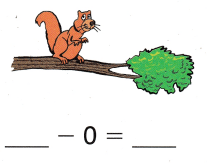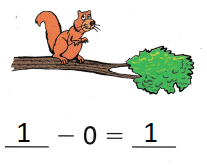Explanation:
1 – 0 = 1
The number subtracted with the 0 the answer remains itself
When a number subtracted by itself the answer is 0

Question 2.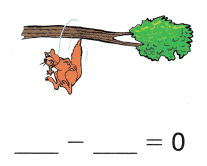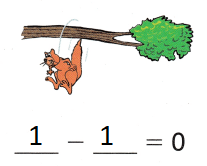Explanation:
1 – 1 = 0
The number subtracted with the 0 the answer remains itself
When a number subtracted by itself the answer is 0

Question 3.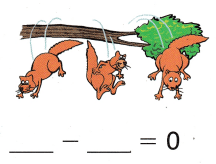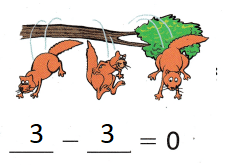Explanation:
3 – 3 = 0
The number subtracted with the 0 the answer remains itself
When a number subtracted by itself the answer is 0

Question 4.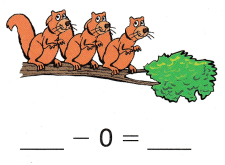Explanation:
3 – 0 = 3
The number subtracted with the 0 the answer remains itself
When a number subtracted by itself the answer is 0

Problem Solving

Write or draw to solve.

Write or draw to solve.

Question 5.
Rashid has 7 fewer toy cars than Ari. Ari has 7 toy cars. How many toy cars does Rashid have?
___ toy cars
0 toy cars
Explanation:
7 – 7 = 0
The number subtracted with the 0 the answer remains itself
When a number subtracted by itself the answer is 0

Texas Test Prep”

Lesson Check

Question 6.
Which number completes the subtraction sentence?
4 – __ = 0
(A) 0
(B) 4
(C) 1
Explanation:
4 – 4 = 0
The number subtracted with the 0 the answer remains itself
When a number subtracted by itself the answer is 0

Question 7.
There are 7 leaves on the tree. The wind blows. 0 leaves fall off the tree. What number sentence shows how many leaves are left?
(A) 7 – 7 = 0
(B) 7 – 0 = 7
(C) 7 – 7 = 1
Explanation:
7 – 0 = 7
The number subtracted with the 0 the answer remains itself
When a number subtracted by itself the answer is 0

Question 8.
Multi-Step Tyler has 9 crayons. He gives some to Al. He gives 3 to Carrie. Now Tyler has 0 crayons. How many crayons did he give to Al?
(A) 0
(B) 3
(C) 6Explanation:
9 – 9 = 0
The number subtracted with the 0 the answer remains itself
When a number subtracted by itself the answer is 0

Question 9.
What is the difference for 4 – 0?
(A) 4
(B) 0
(C) 1